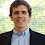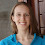### A Simple Proof: Why you will never see an eight sided snowflake

Suppose you take a bunch of equally-sized marbles and squish them from all sides. You'll get one of two shapes:

These configurations are referred to as "rectangular" and "hexagonal":

Certain crystals have a molecular structure similar to these patterns, which is reflected in their macroscopic structure:

A theorem known as the crystallography restriction states that hexagonal symmetry is the most that any crystal can hope to have - you will never see an eight-sided snowflake.

### Formalizing a crystal

This theorem involves only basic trigonometry, but it does require us to most formally state what we mean by a "crystal". (If you know some basic linear algebra, most of this won't be a surprise to you.) The concept we're looking for is a discrete lattice, which has two relevant properties:

1. Every two points are some finite distance apart (i.e. points aren't infinitely close together).
2. The structure repeats along some line, meaning that you can slide the entire thing some finite distance along that line and get back what you started with. Another way of describing this is like an abstract version of addition. Basically, draw two lines which both start and end in the center of some circles. When you place the lines end to end, the last one will still be in the center of some circle. (So "adding" two lines together gets a valid third line.)

Lastly, we need some way of describing what exactly we mean by having a "six-sided" crystal. Just like we can describe "sliding" repetition with addition of lines, we can describe rotational symmetry with rotations of lines.

### Down to earth

As a warm up to our theorem that 6 is the most sides that a crystal can have, let's prove that no crystal has 5 sides.

Suppose we have something with five sides (i.e. a pentagon). If it were a lattice, we could add any two lines together to get a third line. So let's bring the yellow line down as the red one, and add it to the blue one. But this doesn't terminate on a point. So we can't add any two lines together, meaning it can't be a lattice. QED.

The general case is only somewhat more complex. Start with the yellow line, then rotate it by some angle θ to get the blue line. Subtract the yellow line from the blue line to get the green line. If θ is less than 360/6, the green line will be shorter than the yellow line.

We stated in our definition of "discrete lattice" above that every two points must be some finite distance D apart from each other. Phrased differently, every line must have a length of at least D. But we showed above that, if a rotation by θ is legal, we can get smaller and smaller lines - eventually we will get one smaller than D, causing a contradiction.

Therefore, any lattice must not have a rotation smaller than 60 degrees. QED.

So if anyone tries shows you an octagonal snowflake this holiday season, either they're lying, or they violated the laws of math to make an xmas miracle.

### Appendix

I didn't really prove that the green line would be smaller. It's not too long, but it's not terribly interesting, so I'll just leave a link to wikipedia.

Update: I was worried that this would make the post too long, but here's a proof that the green line will be shorter. Starting from O, draw some line out to A. We only care about if the line gets shorter, not its absolute length, so let's just assume that the line $\overline{OA}$ has length one. Rotate $\overline{OA}$ to find point $B$, and subtract $\overline{OB}-\overline{OA}$ to get $C$. Since $\overline{BC}$ and $\overline{OA}$ are parallel, the angles $\angle AOB$ and $\angle OBC$ are equal. By the law of cosines, $\overline{OC}$ has length $\overline{OB}^2 + \overline{BC}^2 - 2 \overline{OB}\cdot\overline{BC}\cdot\cos\theta$. Recalling that $\overline{OA}=\overline{OB}=\overline{BC}=1$, we get that $\overline{OC}=2-2\cos\theta$. If $\theta\lt 60^{\circ}$, then this value will be less than one, meaning that $\overline{OC}\lt\overline{OA}$. So $\theta\geq 60^{\circ}$. QED.

The ugly pictures were created by me. The good-looking pictures courtesy of Wikimedia.

### You might also like

1.Super cool!

2.I'm not sure I follow.

The diagram where you add the blue and yellow vectors seems inaccurate. I don't think the red vector is the sum of the blue and yellow ones; there is no way it could be horizontal since the blue one has some angle and the yellow one doesn't. I suppose if you rotated it after the sum it still works, but its unclear in that diagram.

Also " Start with the yellow line, then rotate it by some angle θ to get the blue line. Subtract the yellow line from the blue line to get the green line. If θ is less than 360/6, the green line will be shorter than the yellow line." It isn't clear at all to me why thats true.

3.@timwizard: you are absolutely right about the red line. Its terminus is in the right place, but not its origin.

I added a brief proof at the end that the green line will be shorter.

4.In addition to the vector addition mistake timwizard pointed out, I think there is another mistake in the last proof. I think OB - OA = CO.

5.Thanks Gina, I have updated the proof at the bottom.

6.Thank's you made it really very clear!!!

7.The inherent structure of our visual perception limits our ability to perceive an object with eight sides all at once. How Information Safeguard Our brain processes visual information in a way that favors simpler shapes.